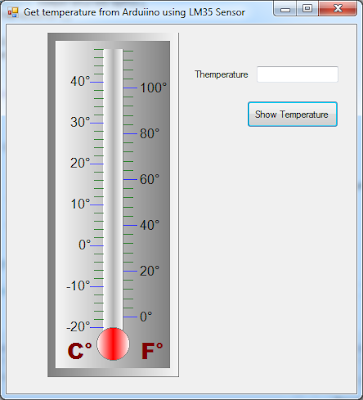# Temperature sensor LM35 in Arduino with CSharp

## 1. Problem Statement

In this tutorial we will learn how use temperature sensor LM35 to get temperature from the environment in Arduino and process the information in C# via the serial Port, Also we will show the temperature in Celsius and Fahrenheit degrees.

## 2. LM35 Precision Centigrade Temperature Sensors

The LM35 is integrated-circuit temperature sensor, whose output voltage is linearly proportional to the Celsius (Centigrade) temperature. The LM35 thus has an advantage over linear temperature sensors calibrated in ° Kelvin, as the user is not required to subtract a large constant voltage from its output to obtain convenient Centigrade scaling. The LM35 does not require any external calibration or trimming to provide typical accuracies of ±1⁄4°C at room temperature and ±3⁄4°C over a full −55 to +150°C temperature range. Low cost is assured by trimming and calibration at the wafer level.. The LM35 is rated to operate over a −55° to +150°C temperature range.
Previously we need to understand couple of things, so to connect it to Arduino, you must connect VCC to Arduino 5V, GND to Arduino GND, and the middle pin you must connect to an Analog Pin, in my case we will use A0(Analog pin). This projects doesn't need any resistor or capacitor between the Arduino and the Sensor, but you need to know that Arduino ADC has a precision of 10 bits. This means:
5V / 2^10 = 5V / 1024, so 0.0049 is our factor. To get the temperature in Celsius from LM35, we calculate like this: Temperature Celsius = (pinA0 * 0.0049)*100

## 3. Sketch for this project

For implement this project we will required the following materials:
• LM35 temperature sensor
• Couple of Wires
• Arduino UNO R3
The next picture show the sketch for this project## 4. Source code to Get Temperature from LM35 in Arduino

Go to Arduino text Area and type de following code, first we define the analog Pin, sensorValue and temperature in Celsius, second we start to open communication for serial, third we get the voltage from LM35 and convert to celsius degree and write in the serial port and get the information from C# and show the temperature.
` #define sensorPin 0 //A0 Pinint sensorValue = 0; float celsius = 0;void setup() {   Serial.begin(9600); //start Serial Port} void loop() {   getTemperature();  //send celsious value to Port and read from C#  Serial.println(celsius);   delay(1000); }void getTemperature(){  //get sensorPin voltage  sensorValue = analogRead(sensorPin);  //convert voltage to celsius degree  celsius = (sensorValue * 0.0049) *100; }`

## 5. Design Project in C# to show temperature in Celsius and Fahrenheit

Design an interface like depicted in next figure, for design the thermometer I used the Old GDI+, also we will add a TextBox to show the temperature get from serial Port and finally one Button to show the temperature in the thermometer.The next code is for button Show temperature, first we verify if the port is open and after get the temperature from serial port which was send by arduino and round this value and send that value to a function which is in charge to draw the current temperature in the thermometer.
` private void btnShowTemperature_Click(object sender, EventArgs e){  try  {     if (serialPort1.IsOpen)     {       //get Temperature from serial port sent by Arduino               int temperature = (int)(Math.Round(Convert.ToDecimal(                          serialPort1.ReadLine()), 0));       txtTemperature.Text = temperature.ToString();       //draw temperature in the thermometer       DrawTemperatureLevel(temperature);     }   }   catch (Exception ex)   {     MessageBox.Show(ex.Message);   }          }        `

## 6. Running the project

First in arduino UNO R3 press the button Upload and then go to visual studio and run the project, second press the button Show Temperature and then it will show the temperature in the thermometer as shown next figure.you can watch the video tutorial on youtube.